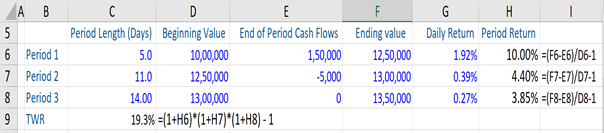## What is “Portfolio Performance?”

Portfolio performance refers to evaluating the performance of an investor’s investment portfolio. It is essentially a process of comparing a portfolio’s return with the return earned on a benchmark portfolio (or one or more other portfolios or indices).

When referring to portfolio performance, five key concepts need to be known:

1. Components of return
2. Total or Holding Period Return (HPR)
3. Absolute and relative performance
4. Rolling returns
5. Time-Weighted Rate of Return (TWR) and Money-Weighted Rate of Return (MWR)

## Key Learning Points

• Portfolio performance evaluates the portfolio performance versus a chosen benchmark or alternative portfolio.
• The four measures of portfolio performance are:
• Total return, which includes income and capital appreciation or loss.
• Absolute and relative performance
• Rolling returns, which involves a multiple time period measurement of investment performance.
• Time Weighted Return (TWR) and Money Weighted Rate of Return (MWR)
• To calculate the time weighted returns, one needs to link each period’s return and multiply the same in a compounding manner.

## Portfolio Performance – the Differing Measures

Total Return: Let’s now look at total return in more detail. It is typically the most comprehensive measure of investment return as it incorporates the two primary components of return that an investor earns from an investment or investment portfolio. These are the interest payments on fixed-income investments, dividends from stocks, or distributions that an investor receives, and capital appreciation realized over a given period of time.

Total return is also known as holding period return in many cases. This refers to the total return earned from an investment or an investment portfolio over the total period for which the asset or the portfolio was held by that investor.

Formula:

HPR = Market price (end period) – Market Price (beginning period) + Income/Market Price (beginning period)

Absolute and Relative Performance: Absolute returns are the actual returns on a security or portfolio. It combines both income generated by the investment and capital appreciation or loss. The investment is measured for a specific time period, and then the absolute performance can be used to compare it to other benchmarks and portfolios to see what the relative performance has been. Relative performance is the difference between absolute return on a security or portfolio and the benchmark return.

There are various types of benchmarks when calculating relative performance. These could be the overall broad market (for example, the S&P 500 in the US) or similar investment funds, or a subset of companies that are comparable.

Rolling Returns: There are several portfolio measurement options and they generally fall into two categories – a single period and a multiple time period measurement. The latter is known as a rolling return measurement. Rolling returns display returns in overlapping cycles, and goes to show the frequency or magnitude of an investment’s good and bad performance periods. By looking at rolling returns, we can get a better appreciation for how an investment’s returns stacked up at any point in time, not through just the last month or quarter or year.

Time Weighted Return (TWR) and Money (Dollar) Weighted Return (MWR): These are two methods or approaches of evaluating portfolio performance vis-à-vis the rate of return on a portfolio. The TWR is the compound rate of growth over a stated evaluation period of the \$1 initially invested in the account. This requires that the account be revalued every time there is an incoming cash inflow or outgoing cash flow. The formula for TWR is:

TWR = (1+r1) * (1+r2)* (1+rn) – 1

*r is equal to the return in different periods.

Regarding MWR, it measures the compound growth rate in all funds investment in the account over the evaluation period. It is also known as the “internal rate of return” or IRR. Essentially, the MWR is the discount rate where the present value of all cash inflows equals the present value of all cash outflows.

MWR = CF0 + CF1/(1+IRR) + CF2/(1+IRR)^2 + CF3/(1+IRR)^3 +….+ CFn/ (1+IRR)^n

## Portfolio Performance – TWR, Example

Shown below, we have a portfolio over three (3) periods with different beginning and ending values, and various cash flows throughout the period. From here, we first calculate the period return (i.e. r1, r2, r3) for each of these periods. The same is calculated as the ending value of a period minus any cash flows in that period divided by the beginning value of the period minus 1. This eliminates the effects of portfolio cash flows on the returns (which are calculated below). Therefore, the calculations are not sensitive to the contributions or withdrawals.

Having stated the above, we now want a rate of return measure which is not sensitive to cash flows and this is where the TWR comes in. If we link each period’s return and multiply them in a compounding manner, we can calculate the TWR (using the formula) for the portfolio across the three periods, which in this case is 19.3%.